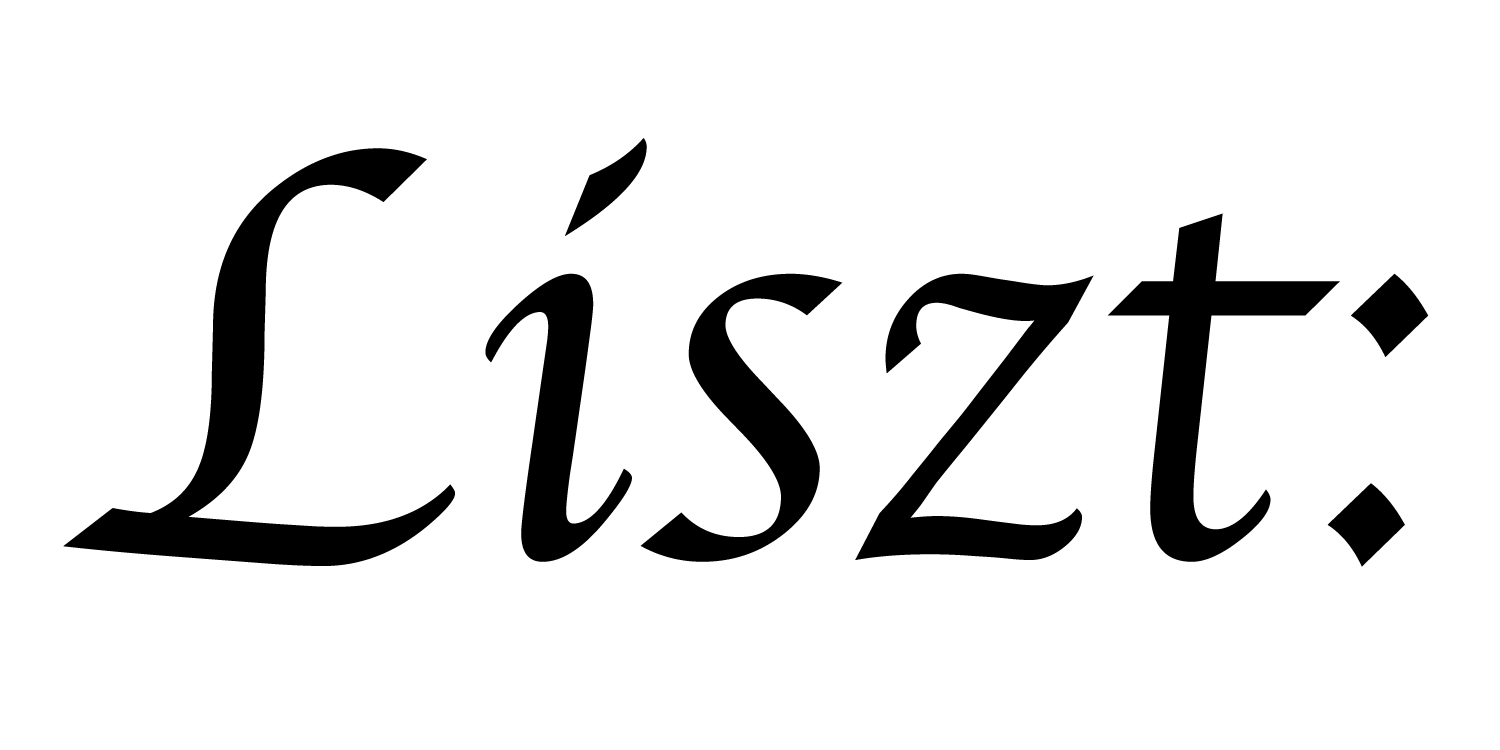# 05: Accessing Neighbors

In order to do useful simulation computations, we need to not just execute computations at each element of a mesh. We also need those computations to access data from neighboring elements. That is, we need some way to access the mesh’s topology inside of our functions.

``````import 'ebb'
local L = require 'ebblib'

local ioOff = require 'ebb.domains.ioOff'
local PN    = require 'ebb.lib.pathname'
local mesh  = ioOff.LoadTrimesh( PN.scriptdir() .. 'bunny.off' )

local vdb   = require('ebb.lib.vdb')
``````

We’ll start this program again the same way, except we’ll load the Stanford bunny mesh instead of the octahedron.

``````local timestep    = L.Constant(L.double, 0.45)
local conduction  = L.Constant(L.double, 1.0)
``````

Here we use an alternative to global variables to define quantities that will be constant over the course of a simulation. By defining these quantities as constants, we allow for Ebb to more aggressively optimize their use in code.

``````mesh.vertices:NewField('t', L.double):Load(0)
``````

We also define a temperature field and change in temperature field. However, if we just ran the simulation with temperature 0 everywhere, nothing would happen.

``````local function init_temperature(idx)
if idx == 0 then return 1000 else return 0 end
end
``````

So instead, we use a Lua function (taking the vertex index in a range `0` to `mesh.vertices:Size()-1`) that returns the temperature that each vertex should be initialized to. We use this function to place a bunch (1000) of units of temperature on the first vertex.

``````local ebb compute_update ( v : mesh.vertices )
var sum_t : L.double = 0.0
var count            = 0

for e in v.edges do
count += 1
end

var avg_t  = sum_t / count
var diff_t = avg_t - v.t
v.d_t = timestep * conduction * diff_t
end

local ebb apply_update ( v : mesh.vertices )
v.t += v.d_t
end
``````

We use two functions to define a step of heat diffusion. The first function computes a change in temperature and the second one applies it. (This may seem strange, but let’s try to understand what the functions are doing before we figure out why there are two of them; we’ll discuss the reason for using two functions in the next example program.)

The first function `compute_update()` loops over the edges of a given vertex `for e in v.edges do ...`, in order to compute the average temperature of the neighboring vertices. Then we simply update the current temperature to be a bit more similar to the average neighboring temperature. This is a pretty standard heat diffusion simulation strategy.

Notice that we were able to loop over the edges of the vertex, and then access the temperature at `e.head.t`. These are two of the forms of neighbor access provided by the triangle mesh domain library. They allow us to access other nearby elements starting from the centered element of the function that was passed in as the parameter.

``````local ebb visualize ( v : mesh.vertices )
vdb.color({ 0.5 * v.t + 0.5, 0.5-v.t, 0.5-v.t })
vdb.point(v.pos)
end
``````

As before, we write a simple visualization function for VDB. This time, we use the color channel to visualize the heat of a point

``````for i=1,360 do
mesh.vertices:foreach(compute_update)
mesh.vertices:foreach(apply_update)

vdb.vbegin()
vdb.frame()
mesh.vertices:foreach(visualize)
vdb.vend()
end
``````

The simulation loop is almost identical to last time. If you run the program with visualization, you should see the heat (in red) spread out over the bunny’s back.

a part of the Liszt project and PSAAP II center at Stanford University